# Inverse - Definition with Examples

The Complete K-5 Math Learning Program Built for Your Child

• 40 Million Kids

Loved by kids and parent worldwide

• 50,000 Schools

Trusted by teachers across schools

• Comprehensive Curriculum

Aligned to Common Core

## Definition of Inverse?

In mathematics, the word inverse refers to the opposite of another operation.

Let us look at some examples to understand the meaning of inverse.

Example 1:

The addition means to find the sum, and subtraction means taking away. So, subtraction is the opposite of addition. Hence, addition and subtraction are opposite operations.  We may say, subtraction is the inverse operation of addition.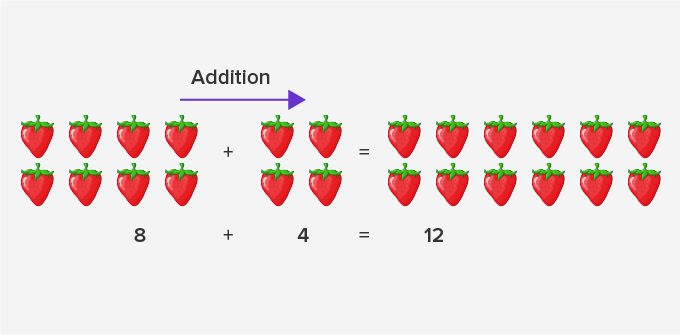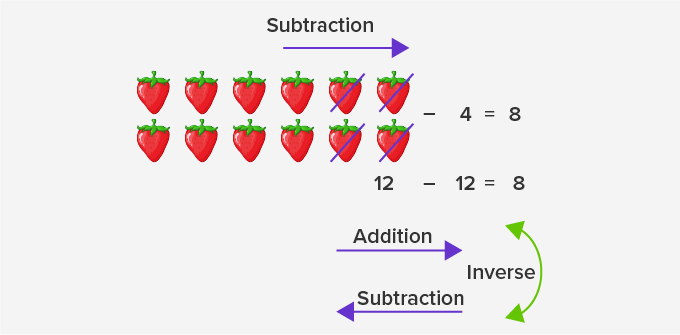Example 2:

However, the division is repeated subtraction. The same number gets subtracted repeatedly.  So, the division is the opposite of multiplication. Hence, multiplication and division are opposite operations. We may say, division is the inverse operation of multiplication.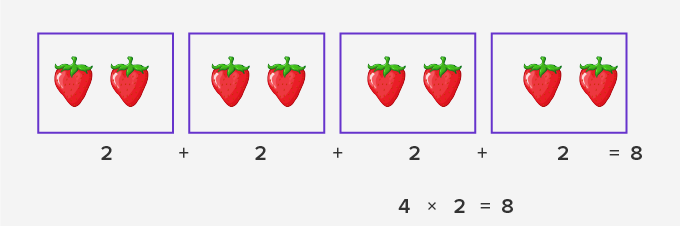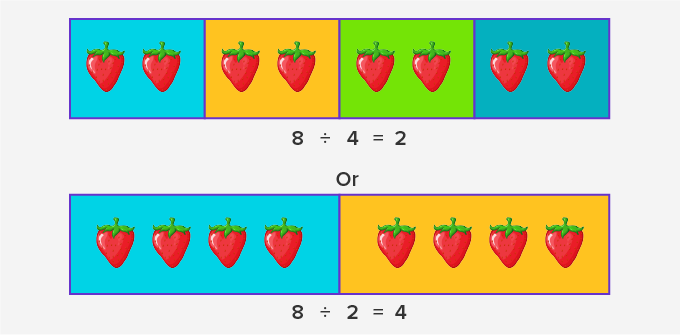So, we see that multiplication and division are inverses of each other.

## Multiplicative Identity:

A multiplicative identity is a number which when multiplied by any non-zero number gives the same number as the product.

For example, if a is any non-zero number, then multiplying a  by 1 gives the product as the number itself.

a × 1 = a

Therefore, 1 is the multiplicative identity.

## Multiplicative Inverse:

A multiplicative inverse is a number which when multiplied by a number gives 1 (multiplicative identity) as the product.

If a is any non-zero number, then multiplying a by what number gives the product as 1?

a × ? = 1

As, aa = 1, we need a number in which a is in the denominators place. We have a in the numerator. So, we keep 1 is the numerator of this number and get 1a.

a × 1a = 1

So, 1a is the multiplicative inverse of a.

Multiplicative inverse is also called a reciprocal.

Reciprocal of a number is obtained by interchanging its numerator and denominator.

The multiplicative inverse of a is 1and multiplicative inverse of a fraction  ab is ba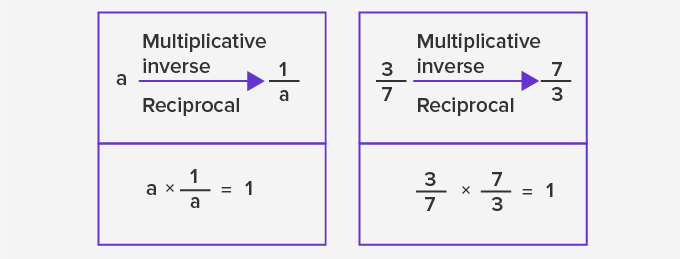Fun Facts Inverse property is also seen in proportion. It is called the inverse proportion. In inverse proportion, when one value increases the other decreases. For example, if the number of machines increases, the time taken to complete the task decreases.

Won Numerous Awards & Honors